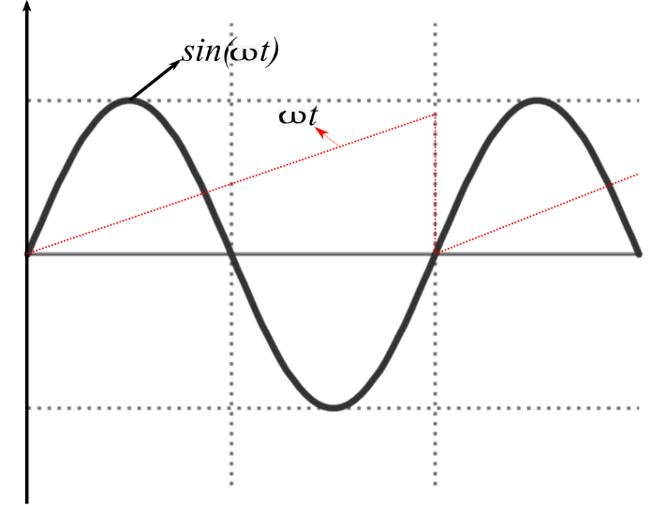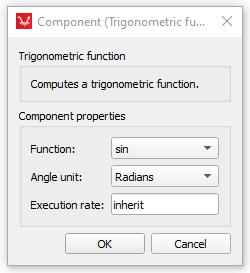# Trigonometric Function

Description of the Trigonometric Function component in Schematic Editor, which computes one of the available trigonometric functions to the input signal.

## Description

The Trigonometric Function component computes one of the available trigonometric functions to the input signal.

Figure 2 illustrates the behavior of the trigonometric function component configured as a sinusoidal function when an input corresponding to the frequency ωt is applied to this component’s input.Figure 2. Sine waveform produced by the trigonometric function component from the input frequencyωt.

## Ports

• Input (in)
• Input signal. When “atan2” is used as a function, this component will display two inputs, which are described as follows:
• Primary input – marked with ( ' ) - The primary input that is used as the x-axis variable of the Cartesian coordinates.
• Secondary input - The secondary input that is used as the y-axis variable of the Cartesian coordinates.
• Supported types: uint, int and real
• Vector support: yes.
• Output (out)
• Result of the selected trigonometric function.
• Supported types: uint, int and real.
• The output type is inherited from the input signal.
• Vector support: yes.
• The vector length is inherited from the input signal

## Properties• Function
• Select the trigonometric function that will be computed. Seven operations are available:
• sin(input): Outputs sine function of the input signal.
• cos(input): Outputs cosine function of the input signal.
• tan(input): Outputs tangent function of the input signal.
• asin(input): Outputs arcsine function of the input signal. This function only exists for input signals in the range between (-1<=input<=1).
• acos(input): Outputs arccosine function of the input signal. This function only exists for input signals in the range between (-1<=input<=1).
• atan(input): Outputs arctangent function of the input signal.
• atan2(input1, input2): Outputs arctangent of the Cartesian coordinates (input1, input2). The result of this function provides an unambiguous value for the angle related to this function inputs.
• Angle unit
• Input angle in operations sin(input), cos(input) and tan(input) can be entered in either radians or degrees.
• Output angle in operations asin(input), acos(input), atan(input) and atan2(input1, input2) can be in either radians or degress.
• Execution rate
• Type in the desired signal processing execution rate. This value must be compatible with other signal processing components of the same circuit: the value must be a multiple of the fastest execution rate in the circuit. There can be up to four different execution rates, but they must all be multiple of the basic simulation timestep. To specify the execution rate, you can use either decimal (e.g. 0.001) or exponential values (e.g. 1e-3) in seconds. Alternatively, you can type in ‘inherit’ in which case the component will be assigned execution rate based on the execution rate of the components it is receiving input from.